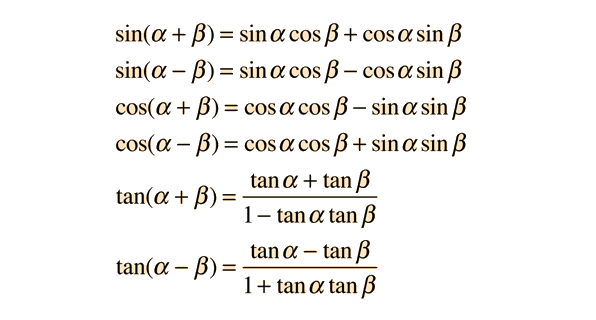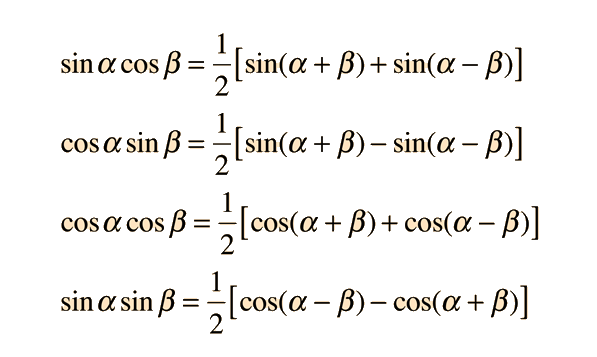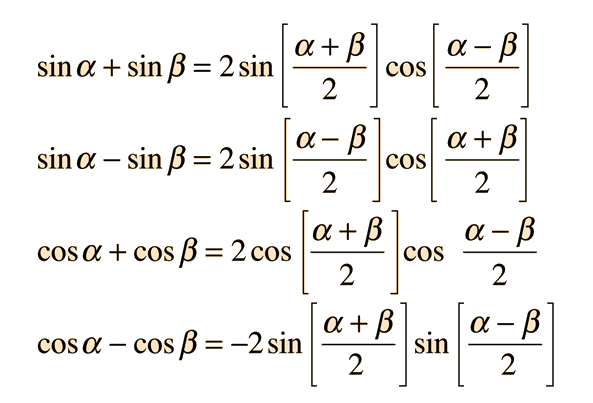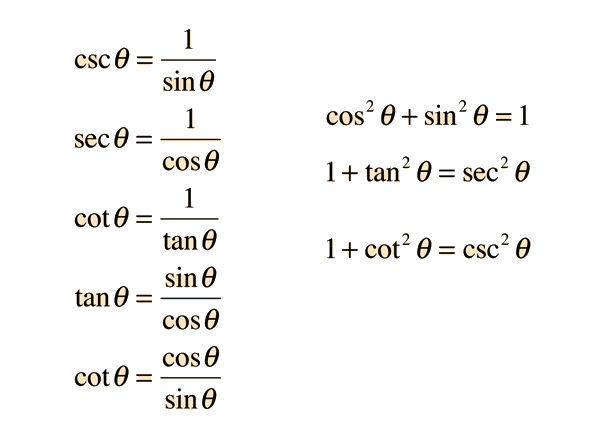# Angle sum and differenceApplications:
 Rutherford scattering Instantaneous electric power Sum and difference frequencies
Index

 HyperPhysics****HyperMath*****Trigonometry R Nave
Go Back

# Product FormulasThese relationships express the product of two sinusoids in terms of the sum of two sinusoids. Sometimes it is desirable to express the sum of two sinusoids in terms of a product of sinusoids, as in the description of modulated sine waves. These relationships are called the superposition relationships.

Index

 HyperPhysics****HyperMath*****Trigonometry R Nave
Go Back

# Superposition RelationshipsIf the sinusoids represent traveling electromagnetic waves and the arguments of the sinusoids are proportional to frequency, then these relationships show that the superposition of two sinusoids will produce components with the sum and difference of the two frequencies.

Index

 HyperPhysics****HyperMath*****Trigonometry R Nave
Go Back

# Fundamental IdentitiesIndex

 HyperPhysics****HyperMath*****Trigonometry R Nave
Go Back